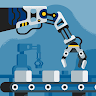Published

# Autonomous Air Sanitization Machine

Autonomous Air Sanitization Machine filter airborne COVID-19 particles and provide virus free air using UV light disinfection process.## Things used in this project

### Hardware components

 ULPA Filter
×1
 UV Light 254nm
×1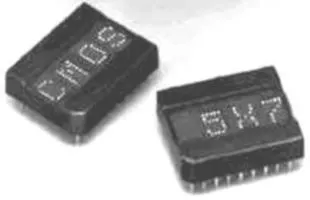Alphanumeric LED Display, Red
×1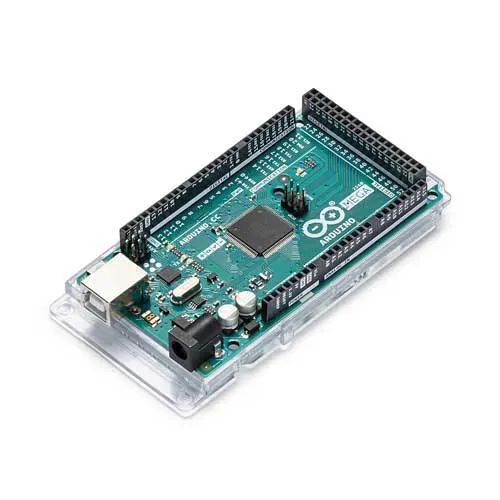Arduino Mega 2560
×1Ultrasonic Sensor - HC-SR04 (Generic)
×3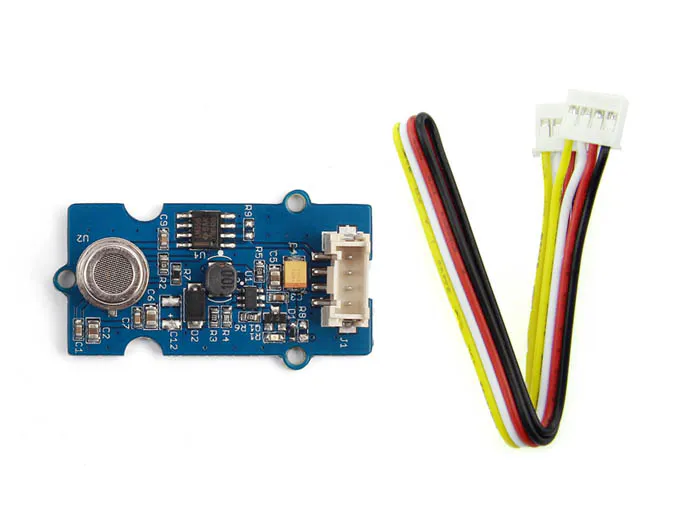Seeed Studio Grove - Air quality sensor v1.3
×1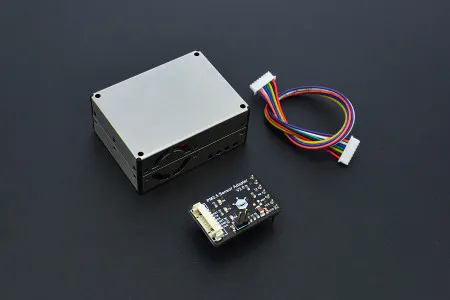DFRobot Air Quality Monitor (PM 2.5, Formaldehyde, Temperature & Humidity Sensor)
×1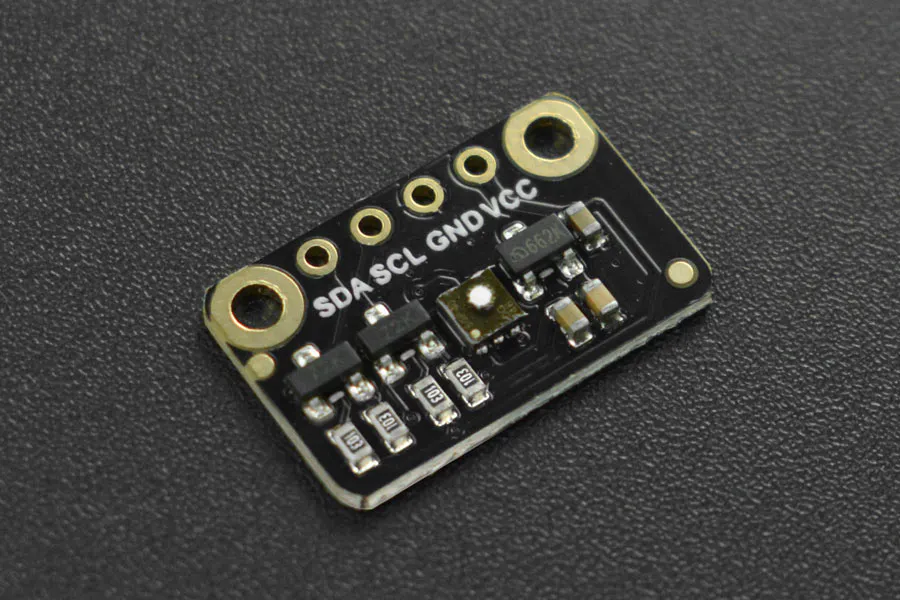DFRobot Fermion: SGP40 Air Quality Sensor (Breakout)
×1Rechargeable Battery, 12 V
×1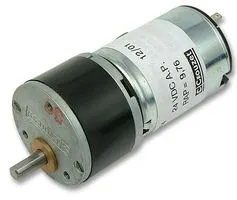Geared DC Motor, 12 V
×4
 wheels
×4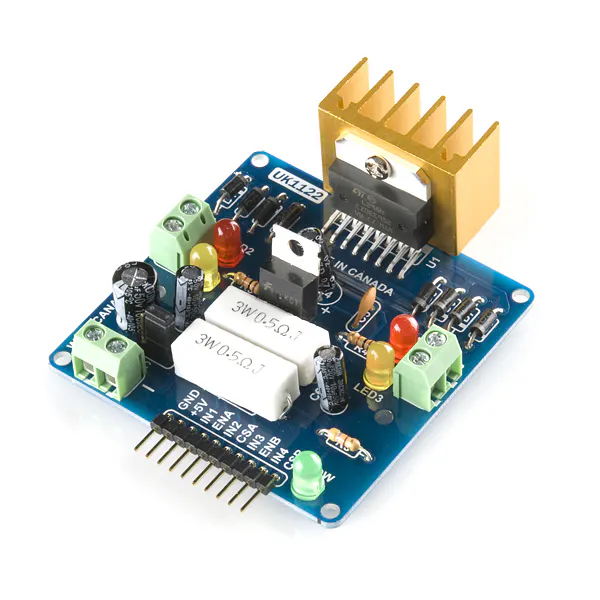SparkFun Dual H-Bridge motor drivers L298
×1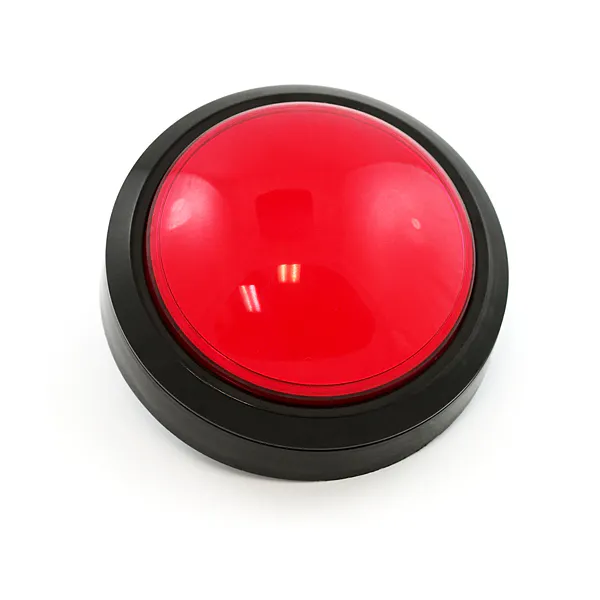SparkFun Big Red Dome Button
×1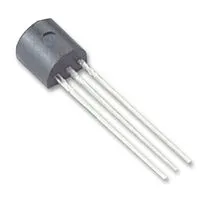General Purpose Transistor NPN
×1
 charging circuit
×1

### Software apps and online servicesArduino IDEMicrosoft Visual Studio 2015

### Hand tools and fabrication machines

 acrylic sheet
 lathe machine

## Schematics

### Circuit Schematics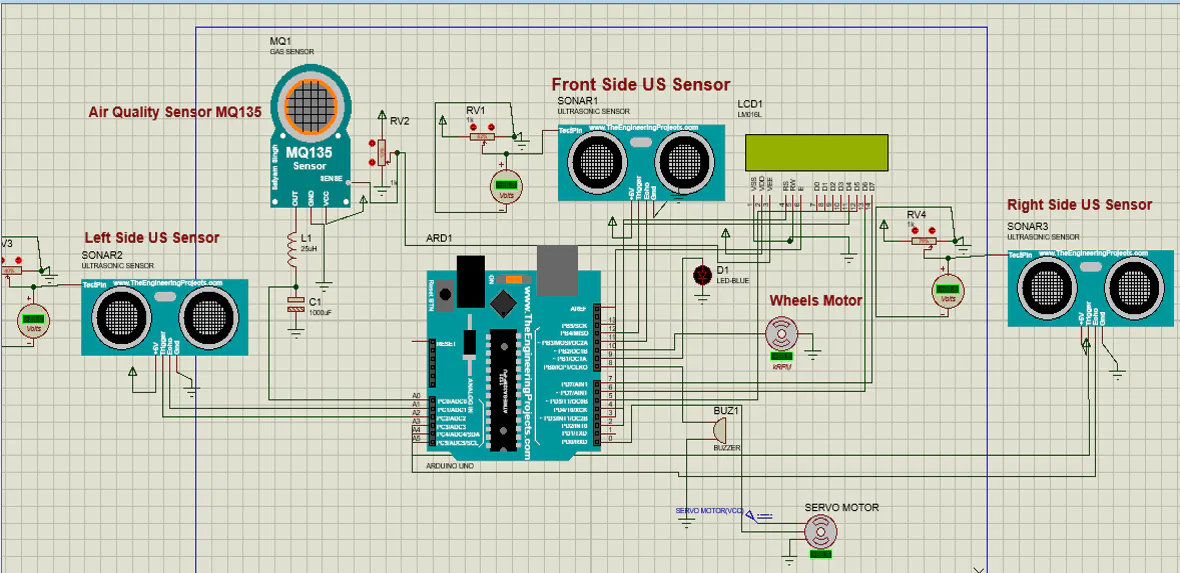### Circuit Schematics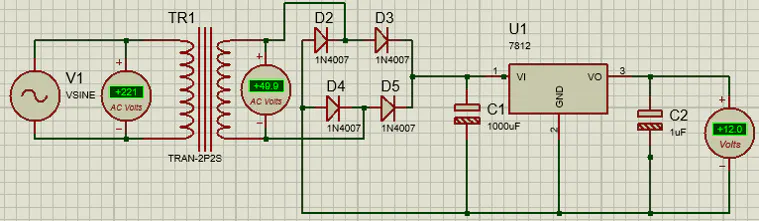## Code

### Moving body code

C/C++
```#include <IRremote.h>// IR remote library
#include <EEPROM.h>// EEPROM pre-installed library
#include<NewPing.h>
NewPing sonarfront (9,10);
/*
***********Left Motor***********
*/
// IN 1
#define ena       4
#define LM_IN1    2
// IN 2
#define LM_IN2    3
/*
************Right Motor************
*/
// IN 3
#define enb       7
#define RM_IN3    5
// IN 4
#define RM_IN4    6
/*
*/
# define RECV_PIN 8// Pin to which IR reciever is connected
IRrecv irrecv(RECV_PIN);// Pass the pin number to the function
decode_results results;// variable results stores the decoded hex values
/*
************HEX code of all the buttons used in the project**************
*/
#define FWD       0xF700FF // go forward(2)
#define LFT       0xF7807F // go left(4)
#define RGT       0xF740BF // go right(6)
#define BWD       0xF7C03F // go backward(8)
#define STOP      0xF720DF // stop(0)
#define RPEAT     0xF7A05F // repeat the stored sequence of movement from the temporary memory(automatically stores) (REPT)
#define DEL       0xF7609F // delete the stored sequence of movement from temporary memory(EQ)
#define PERST     0xF7E01F // copy the sequence from temp. memory to the peramanent memory(EEPROM)
#define PLAYEPROM 0xF710EF // repeat the sequence stored in EEPROM(FASTFWD)
#define RESET     0xF7906F // Resets the Arduino Board(RED)

/*
************Global Variables and Arrays**************
*/

unsigned long int value = 0; // stores the incoming hex value
byte seq = 0; //stores the current number of executed sequences
byte seq_Array;// array to store the movement sequence in terms of integers(1 for FWD, 2 for LEFT and so on..)
//counter for counting the number of times program pass through a movement function(fwd, lft etc.)
int fwd_Counter = -1;
int lft_Counter = -1;
int rgt_Counter = -1;
int bwd_Counter = -1;
int stp_Counter = -1;
//global "current time" variables for different movement functions(fwd, lft etc.)
unsigned long int current_Time0 = 0;// for FWD movement
unsigned long int current_Time1 = 0;// for LEFT movement
unsigned long int current_Time2 = 0;// for RIGHT movement
unsigned long int current_Time3 = 0;// for BWD movement
unsigned long int current_Time4 = 0;// for STOP
//total time spend by the pgm in executing the movement(fwd, lft etc.) for a particular movement counter
unsigned long int total_Fwd_Time;
unsigned long int total_Lft_Time;
unsigned long int total_Rgt_Time;
unsigned long int total_Bwd_Time;
unsigned long int total_Stp_Time;
/*
************Arduino Reset Pin**************
*/
#define RESET_PIN A0
int check =0;
void setup() {
// set mode of the pins as output
for (int i = 2; i <= 7; i++) {
pinMode(i, OUTPUT);
}
// start serial communication
Serial.begin(9600);
// In case the interrupt driver crashes on setup, give a clue
// to the user what's going on.
Serial.println("Enabling IRin");
Serial.println("Enabled IRin");
}
void loop() {
if (irrecv.decode(&results)) {
value = results.value;
Serial.println(value, HEX);
irrecv.resume(); // Receive the next value
delay(200);
}
delay(100);
check_Inst(value);
value=0;
if(sonarfront.ping_cm()<=40 && sonarfront.ping_cm()>0 && check==1)
{
Serial.println("Stopping ultra");
digitalWrite(LM_IN1, LOW);
digitalWrite(LM_IN2, LOW);
digitalWrite(RM_IN3, LOW);
digitalWrite(RM_IN4, LOW);
digitalWrite(ena, LOW);
digitalWrite(enb, LOW);
delay(700);
Serial.println("Going_Forward ultra");
digitalWrite(LM_IN1, HIGH);
digitalWrite(LM_IN2, LOW);
digitalWrite(RM_IN3, HIGH);
digitalWrite(RM_IN4, LOW);
digitalWrite(ena, HIGH);
digitalWrite(enb, HIGH);
}

}
void check_Inst(long int value) {
switch (value) {
case FWD:
go_Forward();
delay(10);
break;
case LFT:
go_Left();
delay(10);
break;
case RGT:
go_Right();
delay(10);
break;
case BWD:
go_Backward();
delay(10);
break;
case STOP:
go_Stop();
delay(10);
break;
case RPEAT:
go_In_Seq();
delay(10);
break;
case DEL:
del_From_Local_Mem();
delay(10);
break;
case PERST:
write_To_Permt_Mem();
delay(10);
break;
case PLAYEPROM:
delay(10);
break;
case RESET:
pinMode(RESET_PIN, OUTPUT);
digitalWrite(RESET_PIN,HIGH);
break;

default:
value = 0;
}
}
void go_Forward() {
movement_Inst_Fwd();
current_Time0 = millis();
int i = seq_Array[(seq - 1)];
switch (i) {
case 2:
// total time elaspsed since Left button is pressed including rest time
total_Lft_Time[lft_Counter + 1] = (current_Time0 - current_Time1);
lft_Counter++;
break;
case 3:
// total time elaspsed since Right button is pressed including rest time
total_Rgt_Time[rgt_Counter + 1] = (current_Time0 - current_Time2);
rgt_Counter++;
break;
case 4:
total_Bwd_Time[bwd_Counter + 1] = (current_Time0 - current_Time3);
bwd_Counter++;
break;
case 5:
total_Stp_Time[stp_Counter + 1] = (current_Time0 - current_Time4);
stp_Counter++;
break;
}
seq_Array[seq] = 1;
seq++;
}
void go_Left() {
movement_Inst_Lft();
current_Time1 = millis();
int i = seq_Array[(seq - 1)];
switch (i) {
case 1:
total_Fwd_Time[fwd_Counter + 1] = (current_Time1 - current_Time0);
fwd_Counter++;
break;
case 3:
total_Rgt_Time[rgt_Counter + 1] = (current_Time1 - current_Time2);
rgt_Counter++;
break;
case 4:
total_Bwd_Time[bwd_Counter + 1] = (current_Time1 - current_Time3);
bwd_Counter++;
break;
case 5:
total_Stp_Time[stp_Counter + 1] = (current_Time1 - current_Time4);
stp_Counter++;
break;
}
seq_Array[seq] = 2;
seq++;
}
void go_Right() {
movement_Inst_Rgt();
current_Time2 = millis();
int i = seq_Array[(seq - 1)];
switch (i) {
case 1:
total_Fwd_Time[fwd_Counter + 1] = (current_Time2 - current_Time0);
fwd_Counter++;
break;
case 2:
total_Lft_Time[lft_Counter + 1] = (current_Time2 - current_Time1);
lft_Counter++;
break;
case 4:
total_Bwd_Time[bwd_Counter + 1] = (current_Time2 - current_Time3);
bwd_Counter++;
break;
case 5:
total_Stp_Time[stp_Counter + 1] = (current_Time2 - current_Time4);
stp_Counter++;
break;
}
seq_Array[seq] = 3;
seq++;
}
void go_Backward() {
movement_Inst_Bwd();
current_Time3 = millis();
int i = seq_Array[(seq - 1)];
switch (i) {
case 1:
total_Fwd_Time[fwd_Counter + 1] = (current_Time3 - current_Time0);
fwd_Counter++;
break;
case 2:
total_Lft_Time[lft_Counter + 1] = (current_Time3 - current_Time1);
lft_Counter++;
break;
case 3:
total_Rgt_Time[rgt_Counter + 1] = (current_Time3 - current_Time2);
rgt_Counter++;
break;
case 5:
total_Stp_Time[stp_Counter + 1] = (current_Time3 - current_Time4);
stp_Counter++;
break;
}
seq_Array[seq] = 4;
seq++;
}
void go_Stop() {
movement_Inst_Stp();
current_Time4 = millis();
int i = seq_Array[(seq - 1)];
switch (i) {
case 1:
total_Fwd_Time[fwd_Counter + 1] = (current_Time4 - current_Time0);
fwd_Counter++;
break;
case 2:
total_Lft_Time[lft_Counter + 1] = (current_Time4 - current_Time1);
lft_Counter++;
break;
case 3:
total_Rgt_Time[rgt_Counter + 1] = (current_Time4 - current_Time2);
rgt_Counter++;
break;
case 4:
total_Bwd_Time[bwd_Counter + 1] = (current_Time4 - current_Time3);
bwd_Counter++;
break;
}
seq_Array[seq] = 5;
seq++;
}
void go_In_Seq(void) {
value = 0;
for (int i = 0; i < (seq + 1); i++) {
int value1 = 0;
value1 = seq_Array[i];
switch (value1) {
case 1:
static int j = 0;
go_Forward_Seq(j);
j++;
break;
case 2:
static int k = 0;
go_Left_Seq(k);
k++;
break;
case 3:
static int l = 0;
go_Right_Seq(l);
l++;
break;
case 4:
static int m = 0;
go_Backward_Seq(m);
m++;
break;
case 5:
static int n = 0;
go_Stop_Seq(n);
n++;
break;
default:
j = 0; k = 0; l = 0; m = 0; n = 0;
}
}
}
void del_From_Local_Mem() {
//set the movement counters to their default values
fwd_Counter = -1;
lft_Counter = -1;
rgt_Counter = -1;
bwd_Counter = - 1;
stp_Counter = - 1;
//set the total movement time to its default value
for (int i = 0; i < 10; i++) {
total_Fwd_Time[i] = 0;
total_Lft_Time[i] = 0;
total_Rgt_Time[i] = 0;
total_Bwd_Time[i] = 0;
total_Stp_Time[i] = 0;
}
// Reset the sequence array(stored movement instructions)
for (int i = 0; i < 50; i++) {
seq_Array[i] = 0;
}
seq = 0;

}
/**********************************************************************************************************
This function copy the data from the arrays to the EEPROM(permanent memory)
************************************************************************************************************/
void write_To_Permt_Mem(){
// total number of movement is stored in a random address i.e, 100
EEPROM.write(100,seq);

//writing the movement sequence
for(int i=0; i<seq; i++){
EEPROM.write(2*i,seq_Array[i]);
}
//storing the time bw two successive movements
for(int i=1; i<seq+1; i++){
if(seq_Array[i-1]==1){
static byte a=0;
EEPROM.write(2*i-1,(total_Fwd_Time[a])/1000);// Note: One location can store maximum value of 255, hence the time is divided by 1000 here. And then multiplied by 1000 while retreiving the data from EEPROM location
a++;
}
else if(seq_Array[i-1]==2){
static byte b=0;
EEPROM.write(2*i-1,(total_Lft_Time[b])/1000);
b++;
}
else if(seq_Array[i-1]==3){
static byte c=0;
EEPROM.write(2*i-1,(total_Rgt_Time[c])/1000);
c++;
}
else if(seq_Array[i-1]==4){
static byte d=0;
EEPROM.write(2*i-1,(total_Bwd_Time[d])/1000);
d++;
}
else if(seq_Array[i-1]==5){
static byte e=0;
EEPROM.write(2*i-1,(total_Stp_Time[e])/1000);
e++;
}
}
}

/**********************************************************************************************************
This function reads the stored sequence from the EEPROM(permanent memory)
************************************************************************************************************/
for(int i=0; i<x+1; i++){
switch(r){
case 1:
movement_Inst_Fwd();
break;
case 2:
movement_Inst_Lft();
break;
case 3:
movement_Inst_Rgt();
break;
case 4:
movement_Inst_Bwd();
break;
case 5:
movement_Inst_Stp();
break;
}
delay((EEPROM.read(i+1))*1000);    // multiplied by thousand because the original time was divided by 1000 while storing in EEPROM.
}
}

/**********************************************************************************************************
These function moves the car in a direction for the time specified/stored in the total_x_time array
************************************************************************************************************/
void go_Forward_Seq(int j) {
//go in forward direction sequence
movement_Inst_Fwd();
delay(total_Fwd_Time[j]);
}
void go_Left_Seq(int k) {
//go in Left direction sequence
movement_Inst_Lft();
delay(total_Lft_Time[k]);
}
void go_Right_Seq(int l) {
//go in right direction sequence
movement_Inst_Rgt();
delay(total_Rgt_Time[l]);
}
void go_Backward_Seq(int m) {
//go in backward direction sequence
movement_Inst_Bwd();
delay(total_Bwd_Time[m]);
}
void go_Stop_Seq(int n) {
//go in Stop sequence
movement_Inst_Stp();
delay(total_Stp_Time[n]);
}
/*********************************************************************************************
These movement instruction are repeated(required) several times in the code
**********************************************************************************************/
void movement_Inst_Fwd(void) {
// forward movement instructions
Serial.println("Going_Forward");
digitalWrite(LM_IN1, HIGH);
digitalWrite(LM_IN2, LOW);
digitalWrite(RM_IN3, HIGH);
digitalWrite(RM_IN4, LOW);
digitalWrite(ena, LOW);
digitalWrite(enb, LOW);
digitalWrite(ena, HIGH);
digitalWrite(enb, HIGH);
check=1;
}
void movement_Inst_Lft(void) {
// Left movement instructions
Serial.println("Going_Left");
digitalWrite(LM_IN1, LOW);
digitalWrite(LM_IN2, HIGH);
digitalWrite(RM_IN3, HIGH);
digitalWrite(RM_IN4, LOW);
digitalWrite(ena, HIGH);
digitalWrite(enb, HIGH);
//delay(3000);// default delay for smooth rotation.
//  digitalWrite(LM_IN1, LOW);
//  digitalWrite(LM_IN2, LOW);
//  digitalWrite(RM_IN3, LOW);
//  digitalWrite(RM_IN4, LOW);
//  digitalWrite(ena, LOW);
//  digitalWrite(enb, LOW);
delay(500);
// NOTE: The minimum delay for RIGHT/LEFT movement is 1S(inluding .5s ON time & .5s OFF time). Hence subtract 1s before repeating this movement
}
void movement_Inst_Rgt(void) {
// Rgt movement instructions
Serial.println("Going_Right");
digitalWrite(LM_IN1, HIGH);
digitalWrite(LM_IN2, LOW);
digitalWrite(RM_IN3, LOW);
digitalWrite(RM_IN4, HIGH);
digitalWrite(ena, HIGH);
digitalWrite(enb, HIGH);
//  delay(3000);// default delay for smooth rotation.
//  digitalWrite(LM_IN1, LOW);
//  digitalWrite(LM_IN2, LOW);
//  digitalWrite(RM_IN3, LOW);
//  digitalWrite(RM_IN4, LOW);
//  digitalWrite(ena, LOW);
//  digitalWrite(enb, LOW);
delay(500);
// NOTE: The minimum delay for RIGHT/LEFT movement is 1S(inluding .5s ON time & .5s OFF time). Hence subtract 1s before repeating this movement
}
void movement_Inst_Bwd(void) {
// Bwd movement instructions
Serial.println("Going_Backward");
digitalWrite(LM_IN1, LOW);
digitalWrite(LM_IN2, HIGH);
digitalWrite(RM_IN3, LOW);
digitalWrite(RM_IN4, HIGH);
digitalWrite(ena, LOW);
digitalWrite(enb, LOW);
digitalWrite(ena, HIGH);
digitalWrite(enb, HIGH);
}
void movement_Inst_Stp(void) {
// Stp movement instructions
Serial.println("Stopping");
digitalWrite(LM_IN1, LOW);
digitalWrite(LM_IN2, LOW);
digitalWrite(RM_IN3, LOW);
digitalWrite(RM_IN4, LOW);
digitalWrite(ena, LOW);
digitalWrite(enb, LOW);
check=0;
}
```

## Credits

### Usama Habib

1 project • 0 followers

### Hafsa Iftikhar

0 projects • 0 followers

### Farwa Rashid

0 projects • 0 followers